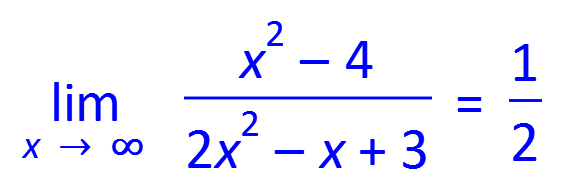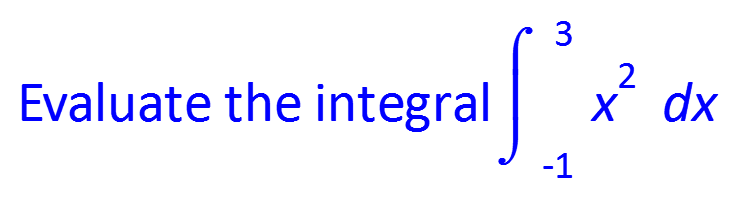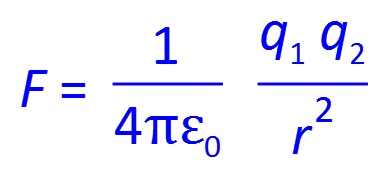# Efofex Type Mathematics – FX Equation

Editmicro » Efofex Type Mathematics – FX Equation

## Efofex Type Mathematics – FX Equation

No one uses equation editors more than mathematics teachers. What they need is high efficiency but most equation editors provide every conceivable feature in an incredibly slow package. FX Equation is different. You just type and FX Equation does all the hard work. No other equation editor comes close to the speed of FX Equation.

FREEGDS

FREEGDS

## Description

FX Equation lets you create equations at the speed of type. Our new FX AutoQuate technology creates an incredibly productive equation-writing environment. You can still create equations using “point and click” but common equations can be produced amazingly fast.

#### Just Type

FX Equation is renowed for it’s extremely high speed equation entry. Most equations can just be “typed” and FX Equation will interpret what you have typed, putting everything in the correct place. For example, typing (x+7)/4×2 will automatically produce this equation. No point and click, just type.

#### There Are Limits!

FX Equation’s “type what you want” paradigm has some limits. In particular, we have limited what it can produce to be representative of the needs of secondary school mathematics and physics teachers. There are some restrictions, but not as many as you might think.

lim(x->inf) (x2-4)/(2×2-x+3) = 1/2#### Seriously Clever

Our FX Autoquate technology lets you just type and FX Equation will automatically decide which bits are equations and which bits are normal text. You don’t have to “do” anything except type. This lets you stop thinking about “how” and concentrate on what you want to write.

Evaluate the integral int(-1,3) x2 dx#### Not Just Mathematics

FX Equation is just at good at rendering the sorts of equations that physics teachers use regularly. This makes it a very useful addition to the FX SciencePack subscription.

F= 1/(4piep\0) (q\1 q\2)/r2